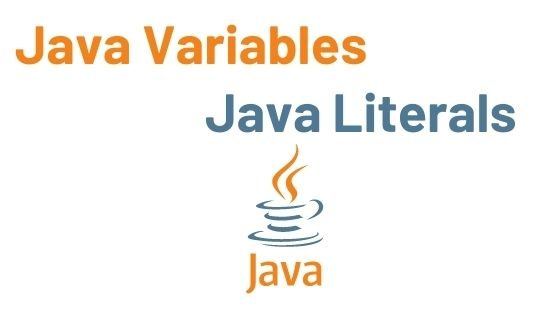## Java Variables | Java Literals | Java Tutorial

In this tutorial, we will learn about variables in java and literals in java.

A variable is a type of named storage that our programs can access. The specific type of each variable in Java determines the size and layout of the memory of the variable, the range of values that can be stored in that memory, and the set of operations that can be applied.

• Variables are data storage containers.
• The term “variable” refers to the name of a memory location.
• Each variable should have a unique name to indicate the storage area (identifier).

Example:

```int n = 5;
float number = 5.99f;
char charVariable = 'D';
boolean booleanVariable = true;
String stringVariable = "Hello"; ```

There are three types of variables in Java:

Contents

## Local Variable Example

```public class Demo {
public void Sample() {
int value = 10;
value += 5;
System.out.println("Value is : " + value);
}

}
class Main{
public static void main(String args[]) {
Demo demo = new Demo();
demo.Sample();
}
}```

Output:

## Instance Variable Example

```public class Student
{

public String name;

private int marks;

public Student (String studentName) {
name = studentName;
}
public void setMarks(int studentMarks) {
marks = studentMarks;
}

public void printStudentInf() {
System.out.println("Name: " + name );
System.out.println("Marks:" + marks);
}

public static void main(String[] args) {
Student StudentOne = new Student("Sagar");
Student StudentTwo = new Student("Avdhesh");
Student StudentThree = new Student("Suraj");

StudentOne.setMarks(70);
StudentTwo.setMarks(80);
StudentThree.setMarks(90);

StudentOne.printStudentInf();
StudentTwo.printStudentInf();
StudentThree.printStudentInf();

}
}```

Output:

## Static Variable Example

```public class Student {

private static int Stipend;

public static final String Company = "Java Developer ";

public static void main(String args[]) {
Stipend = 5000;
System.out.println(Company + "stipend:" + Stipend);
}
}```

Output:

## Java Literals

Literals are data that are used to represent fixed values. They can be directly used in the code. A literal can be a number, a character, or a string, character.

```int x = 10;
float y = 5.5;
char z = 'S';```

Here, 10, 5.5, and ‘S’ are literals.

1: Integer Literals

An integer literal is a numeric value (associated with numbers) that does not contain any fractional or exponential components.

In Java, there are four types of integer literals.

Binary literals in Java begin with 0b, octal literals with 0, and hexadecimal literals with 0x.

Example:

2: Floating-point Literals

An integer part, a decimal point, a fractional part, and an exponent part comprise a floating-point literal. Floating point literals can be represented in either decimal or exponential form.

• A floating-point literal is a numeric literal with a fractional or exponential form.

Example:

```   class Sample {

static void Main(string[] args) {

// float
float x = 3.89f;
Console.WriteLine(x);
// float
float y = 3.14564f;
Console.WriteLine(y);
}
}
```

3: Character Literals

Character literals are character expressions with constant values that are embedded in a Java program. Java characters are 16-bit Unicode characters with values ranging from 0 to 65535. In Java, character literals are represented by a single quote, the character, and a closing single quote ( ‘a’, ‘7’).

instance variable in java, static variable in java, types of variables in java, local variable in java, class variable in java, instance variable in java, static variable in java, types of variables in java, local variable in java, class variable in java , instance variable in java, static variable in java, types of variables in java, local variable in java, class variable in java , instance variable in java, static variable in java, types of variables in java, local variable in java, class variable in java , instance variable in java, static variable in java, types of variables in java, local variable in java, class variable in java , instance variable in java, static variable in java, types of variables in java, local variable in java, class variable in java , instance variable in java, static variable in java, types of variables in java, local variable in java, class variable in java , instance variable in java, static variable in java, types of variables in java, local variable in java, class variable in java , instance variable in java, static variable in java, types of variables in java, local variable in java, class variable in java

• Escape sequences can also be used as a character literal. For example, \b (for backspace), \t (for tab), \n ( for new line), and so on.

4: String literals

In Java, a string literal is a sequence of characters from the source character set that Java programmers use to populate string objects or display text to the user. These characters, which are enclosed within two quotation marks (” “), could be letters, numbers, or symbols of any kind.

```String x = "DevelopersDome";
String y = "Java Android development";```

5: Boolean Literals

variables in java,variables in java,variables in java,variables in java,variables in java,variables in java,variables in java,variables in java

True or false are the only two values that Boolean literals can represent. In Java, a value of 1 is assumed to be true, while a value of 0 is assumed to be false.

You Might Also Like:

Features of Java | Java Tutorial

Java Basic Syntax | Java Tutorial

Difference between JDK, JRE, JVM | Java Tutorial

• We hope that this article will assist you in understanding all about Java Variables, and Java Literals We have concentrated on making a basic, meaningful, and easy-to-learn guide to the concepts. Still, if you have any problems regarding this, please post them in the comment section, and we will be glad to assist you.

java variables,java variables,java variables,java variables,java variables